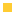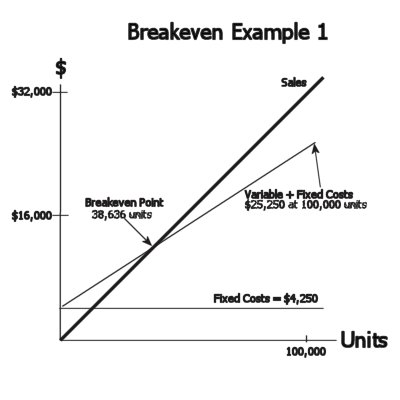continuedIntroduction 3. Break-Even Analysis Break-even analysis is an easy "quantitative" way to analyze opportunities.  It can also be used to compare different solutions. It gives us a way to evaluate opportunities on paper before we invest our resources.  It shows us clearly how many units we have to sell before we will recover our costs. We can create several break-even charts for the same opportunity varying the costs and sales numbers to help us see what might happen with different outcomes.FactorsBreak-evenDatabaseFAQ's
 Central to break-even analysis is the formula:     B-E sales = Price Each*Units = Fixed Costs + Variable Costs which means that we "break-even" when we sell enough units to cover our fixed costs plus our variable costs. Another formula provides the slope of the total cost line:   Slope = Total Variable Costs / Total Quantity A desirable, lower slope number provides better return once we pass B-E sales. The B-E chart is created by drawing the "Sales" line at a 45o angle and creating a vertical axis and a horizontal axis based on the total sales expected for the total number of units sold.  Once we have the \$ on the vertical axis and the units on the horizontal axis we can add the line for "Fixed Costs" and then draw the "Variable Costs" from the "Fixed Cost" line and see where it intersects the "Sales" line.  This is the B-E point.
• 3.1 Example 1 - M Class First Aid Kit Strap
In this example the customer wants to order
100,000 units that we think we can sell for \$0.32 per unit after we invest \$1,000 in tooling; \$2,000 in engineering; \$250 in qualification and \$1,000 in admin cost for a total fixed cost of \$4,250.   We estimate our variable costs at \$0.21 per unit.
 Cost Element Cost \$ Tooling (Fixed) \$1000 Engineering (Fixed) \$2000 Administrative (Fixed) \$1000 Qualification (Fixed) \$250 Material (Variable) \$0.06 each Labor (Variable) \$0.04 each Equipment (Variable) \$0.11 each

B-E sales is
38,636 units
Slope of the variable cost line is
0.21• 3.2 Example 2 - Wire Wrap
In this example the customer wants to order
30,000 units that we think we can sell for \$0.20 per unit after we invest \$500 in tooling; \$1,000 in engineering for a total fixed cost of \$1,500.   We estimate our variable costs at \$0.07 per unit.
 Cost Element Cost \$ Tooling (Fixed) \$500 Engineering (Fixed) \$1000 Material (Variable) \$0.01 each Labor (Variable) \$0.03 each Equipment (Variable) \$0.03 each

B-E sales is
11,538 units
Slope of the variable cost line is
0.07Test Your PK Absorption 1. Why is it a good idea to use break-even analysis? 2. What does break-even analysis show us? 3. What should we do if the break-even quantity is higher than the customer wants to order?